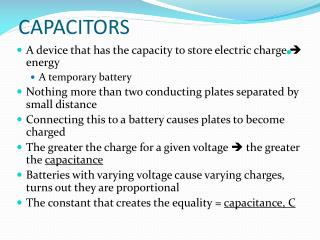DownloadDownload PresentationCAPACITORS

# CAPACITORS

Download Presentation## CAPACITORS

- - - - - - - - - - - - - - - - - - - - - - - - - - - E N D - - - - - - - - - - - - - - - - - - - - - - - - - - -
##### Presentation Transcript

1. CAPACITORS • A device that has the capacity to store electric charge  energy • A temporary battery • Nothing more than two conducting plates separated by small distance • Connecting this to a battery causes plates to become charged • The greater the charge for a given voltage  the greater the capacitance • Batteries with varying voltage cause varying charges, turns out they are proportional • The constant that creates the equality = capacitance, C

2.  C = q / V • Unit: C / V = farad, F (named after Michael Faraday) • Q = magnitude of charge on either plate • V = voltage difference between plates • 1 F capacitor has a huge capacitance • Large capacitance = large ability to store charge  energy

3. Parallel Plate Capacitor • Only type we consider • Two parallel plates of area, A separated by distance, d • As charge is “pulled” from one plate and “placed” on the other because of the battery and E-field is created within plates • We say the E-field stores energy

4. Parallel Plate Capacitor – Cont. • To calculate capacitance:  C = ε0 A / d • Where ε0 = permittivity of free space = 8.85 x 10-12 C2/N m2 • A fundamental constant • As A increases, the surface area increases = more room to store charge • Therefore C increases • The closer the plates are together, d decreases, the larger the capacitance

5. A computer keyboard uses variations in capacitance to “know” whether a key is depressed • The musical instrument, Theremin, uses an antenna as one plate and a hand as another • Zeppelin's Whole Lotta Love • http://www.youtube.com/watch?v=w5qf9O6c20o

6. Electrical Energy Storage • Consider a charged parallel plate • Imagine transferring a small amount of charge, Δq from one plate to the other • Charge must be moved across V, takes energy  ΔU = (Δq) V • As more charges are moved, more work is done  more energy stored

7. Recall: C = q / V  V = q / C • Voltage increases linearly with charge • Total energy, U = q Vave= q (V1 + V2)/2 = ½ q V •  U = ½ q V • Using definition of capacitance, it’s possible to create 2 other equivalent formulas • Energy stored is used in cameras with flash • Defibrillators also use capacitors • Capacitors store charge for extended periods of time and can be quite dangerous